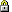## FARTSSIE v2.5 [Software]

Dear all,

up to v2.4 of 14 March 2019 FARTSSIE’s -sheet contained two boxes for reference-scaling. The sample size was wrong because no analytical solution for power exists and simulations are required instead.

In v2.5 of 13 October 2020 Dave deleted the boxes and suggests to install PowerTOST. He gives in two boxes the arguments for PowerTOST’s functions sampleN.scABEL() for Average Bioequivalence with Expanding Limts (EMA and many others, Health Canada) and sampleN.NTIDFDA() for the FDA’s reference-scaling for NTIDs.
However, in the former don’t use the argument regulator="FDA" as he suggests, since RSABE  ABEL. Not only that the regulatory constants are different (≈0.8925742 vs 0.760), these are different approaches (upper limit of the linearized criterion ≤0 vs expansion of the BE limits).

library(PowerTOST) # show the regulatory conditions reg_const(regulator = "EMA") EMA regulatory settings - CVswitch            = 0.3 - cap on scABEL if CVw(R) > 0.5 - regulatory constant = 0.76 - pe constraint applied reg_const(regulator = "HC") HC regulatory settings - CVswitch            = 0.3 - cap on scABEL if CVw(R) > 0.57382 - regulatory constant = 0.76 - pe constraint applied reg_const(regulator = "FDA") FDA regulatory settings - CVswitch            = 0.3 - no cap on scABEL - regulatory constant = 0.8925742 - pe constraint applied

Use the function sampleN.RSABE() instead. Examples with comments at the end.

Since in the survey 20% of participants reported to never update their software: Not a good idea.

library(PowerTOST) sampleN.scABEL(CV = 0.6, design = "2x2x4", regulator = "EMA") # correct +++++++++++ scaled (widened) ABEL +++++++++++             Sample size estimation    (simulation based on ANOVA evaluation) --------------------------------------------- Study design: 2x2x4 (4 period full replicate) log-transformed data (multiplicative model) 1e+05 studies for each step simulated. alpha  = 0.05, target power = 0.8 CVw(T) = 0.6; CVw(R) = 0.6 True ratio = 0.9 ABE limits / PE constraint = 0.8 ... 1.25 EMA regulatory settings - CVswitch            = 0.3 - cap on scABEL if CVw(R) > 0.5 - regulatory constant = 0.76 - pe constraint applied Sample size search  n     power 30   0.7851 32   0.8101 sampleN.scABEL(CV = 0.6, design = "2x2x4", regulator = "HC") # correct +++++++++++ scaled (widened) ABEL +++++++++++             Sample size estimation (simulations based on intra-subject contrasts) ---------------------------------------------- Study design:  2x2x4 (full replicate) log-transformed data (multiplicative model) 1e+05 studies for each step simulated. alpha  = 0.05, target power = 0.8 CVw(T) = 0.6; CVw(R) = 0.6 True ratio = 0.9 ABE limits / PE constraint = 0.8 ... 1.25 HC regulatory settings - CVswitch            = 0.3 - cap on scABEL if CVw(R) > 0.57382 - regulatory constant = 0.76 - pe constraint applied Sample size search  n     power 24   0.7505 26   0.7851 28   0.8118 sampleN.scABEL(CV = 0.6, design = "2x2x4", regulator = "FDA") # wrong +++++++++++ scaled (widened) ABEL +++++++++++             Sample size estimation (simulations based on intra-subject contrasts) ---------------------------------------------- Study design:  2x2x4 (full replicate) log-transformed data (multiplicative model) 1e+05 studies for each step simulated. alpha  = 0.05, target power = 0.8 CVw(T) = 0.6; CVw(R) = 0.6 True ratio = 0.9 ABE limits / PE constraint = 0.8 ... 1.25 FDA regulatory settings - CVswitch            = 0.3 - no cap on scABEL - regulatory constant = 0.8925742 - pe constraint applied Sample size search  n     power 16   0.7017 18   0.7476 20   0.7813 22   0.8071 sampleN.RSABE(CV = 0.6, design = "2x2x4") # correct ++++++++ Reference scaled ABE crit. +++++++++            Sample size estimation --------------------------------------------- Study design: 2x2x4 (4 period full replicate) log-transformed data (multiplicative model) 1e+05 studies for each step simulated. alpha  = 0.05, target power = 0.8 CVw(T) = 0.6; CVw(R) = 0.6 True ratio = 0.9 ABE limits / PE constraints = 0.8 ... 1.25 FDA regulatory settings - CVswitch            = 0.3 - regulatory constant = 0.8925742 - pe constraint applied Sample size search  n     power 16   0.67580 18   0.72735 20   0.76531 22   0.79589 24   0.81947

Dif-tor heh smusma 🖖Helmut SchützThe quality of responses received is directly proportional to the quality of the question asked. 🚮
Science QuotesThread lockedIng. Helmut Schütz